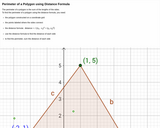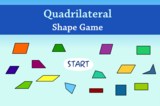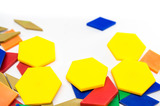Updating search results...

# 7 Results

View
Selected filters:
• polygonConditional Remix & Share Permitted
CC BY-NC-SA
Rating
0.0 stars

In this lesson students explore polygons and sort them by attributes.&nbsp;

Subject:
Mathematics
Material Type:
Activity/Lab
Lesson Plan
Author:
Drew Polly
07/25/2020Rating
0.0 stars

A web page and interactive applet show how to compute the perimeter of a polygon. A polygon is shown that can be resized by dragging its vertices. As you drag, the perimeter is continuously recalculated. Text on the page explains that the perimeter is the sum of the sides. For those who prefer it, in a formula that is given. Applet can be enlarged to full screen size for use with a classroom projector. This resource is a component of the Math Open Reference interactive geometry reference book project at http://www.mathopenref.com.

Subject:
Geometry
Mathematics
Material Type:
Simulation
Provider:
Math Open Reference
Author:
John Page
05/07/2019Unrestricted Use
CC BY
Rating
0.0 stars

Definition of perimeter of polygon, list of items needed when calculating perimeter of polygon on coordinate plane; image of polygon on coordinate plane with additional information in hotspots. Detailed example of calculation of perimeter.

Subject:
Geometry
Mathematics
Material Type:
Interactive
Provider:
Michigan Virtual
07/19/2019Rating
0.0 stars

Students will practice their knowledge of polygons by sorting them using a Carroll Diagram. This interactive game can be used with a whole group or small group of students to reinforce the concept of quadrilaterals as polygons. This whiteboard activity gives instant feedback if students sort incorrectly.

Subject:
Mathematics
Material Type:
Activity/Lab
Provider:
K-5 Math Teaching Resources LLC
Author:
K-5 Math Teaching Resources LLC
02/26/2019Rating
0.0 stars

In this interactive math game, students will find the different type of quadrilaterals for each level of play. They will find quadrilaterals, parallelograms, square, rectangles, rhombus, and trapezoids.

Subject:
Mathematics
Material Type:
Interactive
Provider:
Sheppard Software
02/26/2019Unrestricted Use
CC BY
Rating
0.0 stars

This resource is from Tools4NCTeachers.&nbsp;&nbsp;This task may be&nbsp;used for instruction or assessment.&nbsp;Remix this resource to include extension ideas or addtional tasks.

Subject:
Mathematics
Material Type:
Activity/Lab
Assessment
Formative Assessment
Author:
DAWNE COKER
06/09/2020Unrestricted Use
CC BY
Rating
0.0 stars

This resource is from Tools4NCTeachers.In this lesson, students explore attributes of polygons in order to create 2D shapes using given attributes.&nbsp; Students need some basic background knowledge of the attributes of polygons, including angles, sides, vertices, and right angles.&nbsp;&nbsp;Remix this lesson to include sample student work... Sharing is caring :)

Subject:
Mathematics
Material Type:
Activity/Lab
Lesson
Lesson Plan
Author:
DAWNE COKER